# ggblanket

## How ggblanket works

To simplify ggplot2 visualisation, ggblanket provides:

1. Over thirty gg_* wrapper functions
2. A single col argument to colour and fill by a variable
3. A pal argument to customise colours
4. A facet argument to facet by a variable
5. An additional facet2 argument to facet by a 2nd variable
6. Prefixed arguments to customise titles, scales, guides, and faceting
7. Unspecified x/y/col titles converted to sentence case
8. Ability to set the theme, including to provided themes
9. Pretty defaults for symmetry
11. Ability to add multiple geom_* layers
12. Other key differences to ggplot2
``````library(dplyr)
library(ggplot2)
library(ggblanket)
library(patchwork)

penguins2 <- palmerpenguins::penguins |>
mutate(sex = stringr::str_to_sentence(sex)) |>
tidyr::drop_na(sex)``````

### 1. Over thirty gg_* wrapper functions

Each `gg_*` function wraps a ggplot2 `ggplot(aes(...))` function with the applicable ggplot2 `geom_*()` function. All aesthetics are placed directly in the `gg_*` function: they are not within a `ggplot2::aes` function.

``````# ggplot2
penguins2 |>
ggplot() +
geom_point(aes(x = flipper_length_mm,
y = body_mass_g))``````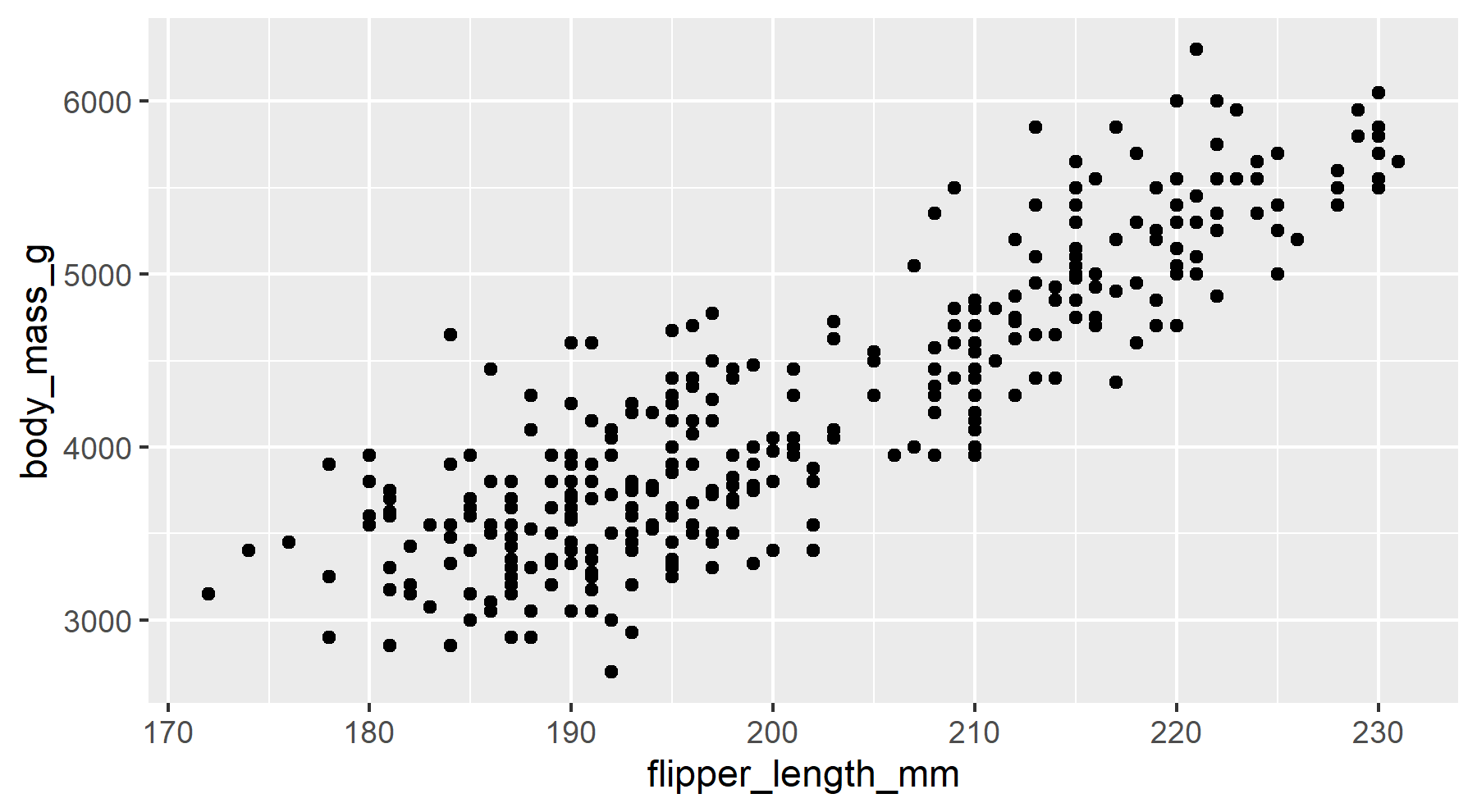``````# ggblanket
penguins2 |>
gg_point(
x = flipper_length_mm,
y = body_mass_g)``````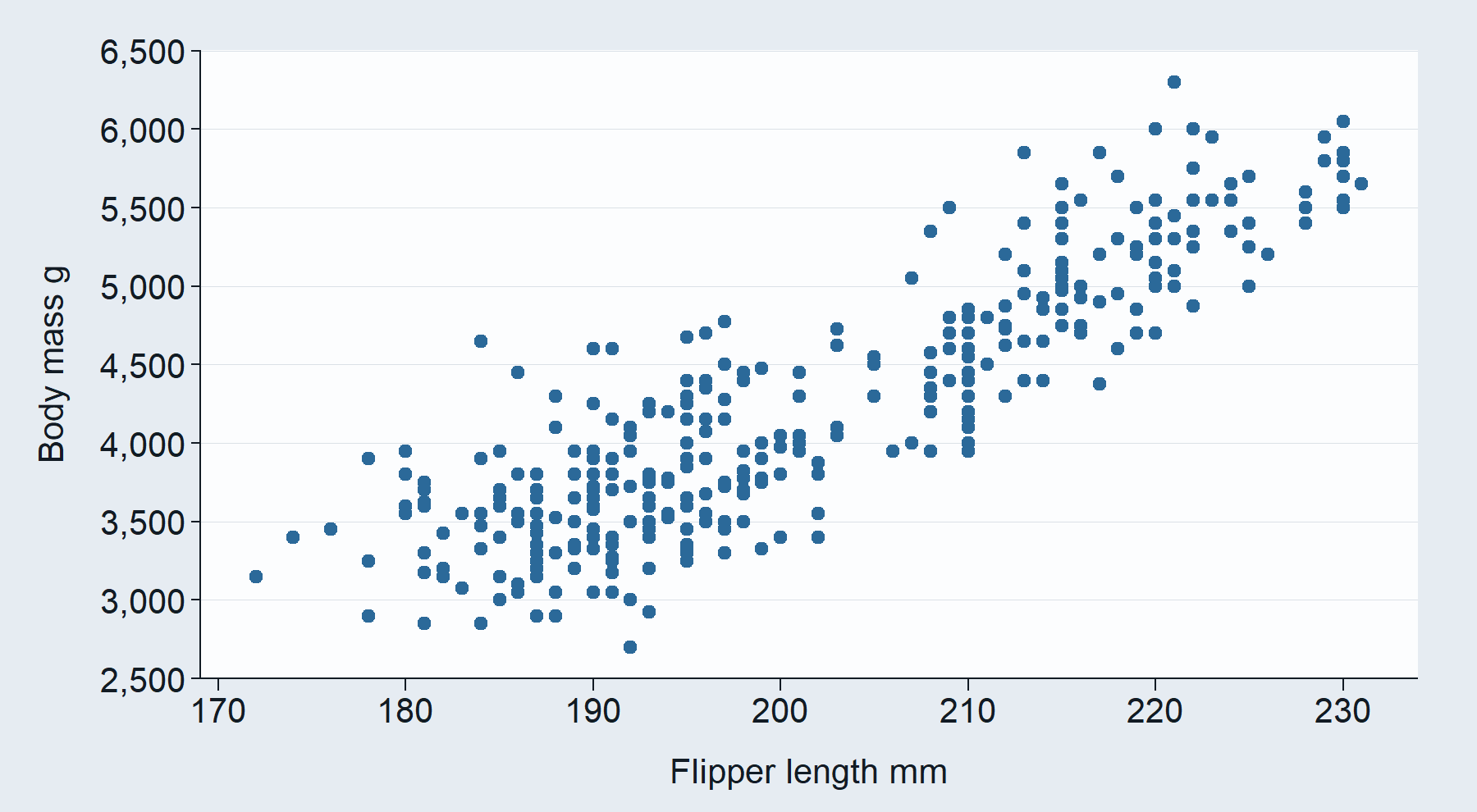### 2. A single col argument to colour and fill by a variable

The colour and fill aesthetics of ggplot2 are merged into a single concept represented by the `col` argument. This argument means that everything should be coloured according to it, i.e. all points, lines and polygon interiors.

``````# ggplot2
p1 <- penguins2 |>
ggplot() +
geom_point(aes(x = flipper_length_mm,
y = body_mass_g,
colour = species))

p2 <- penguins2 |>
ggplot() +
geom_density(aes(x = flipper_length_mm,
fill = species)) +
labs(fill = "Species")

p1 / p2``````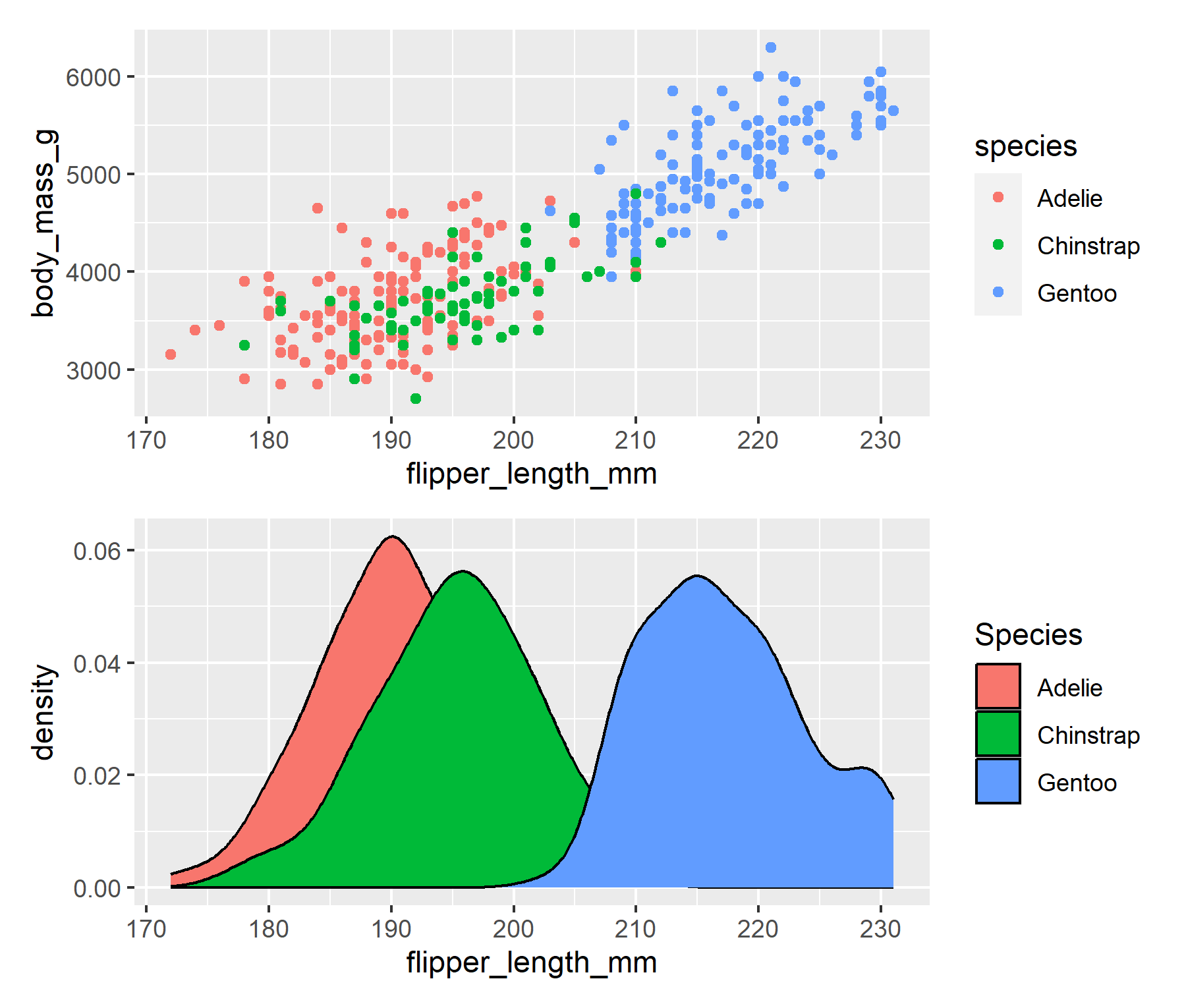``````# ggblanket
p1 <- penguins2 |>
gg_point(
x = flipper_length_mm,
y = body_mass_g,
col = species)

p2 <- penguins2 |>
gg_density(
x = flipper_length_mm,
col = species)

p1 / p2``````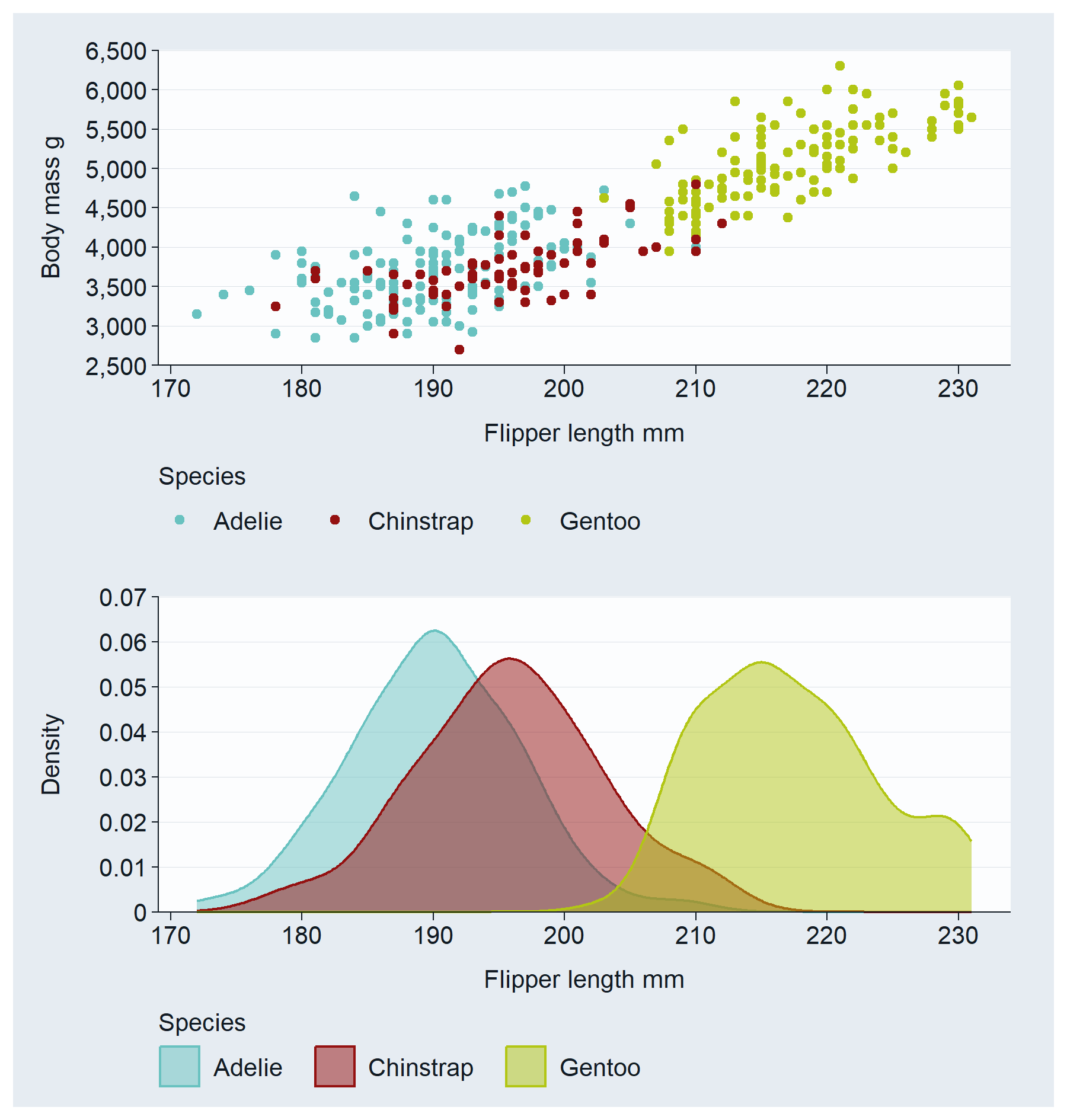### 3. A pal argument to customise colours

The `pal` argument is used to customise the colours of the geom. A user can provide a vector of colours to this argument. It can be named or not. It works in a consistent way - regardless of whether a `col` argument is added or not. A named palette can be used to make individual colours stick to particular values.

``````# ggplot2
p1 <- penguins2 |>
ggplot() +
geom_histogram(aes(x = body_mass_g),
fill = "#1B9E77")

p2 <- penguins2 |>
ggplot() +
geom_jitter(aes(x = species,
y = body_mass_g,
colour = sex)) +
scale_colour_manual(values = c("#2596be", "#fc7c24"))

p1 / p2``````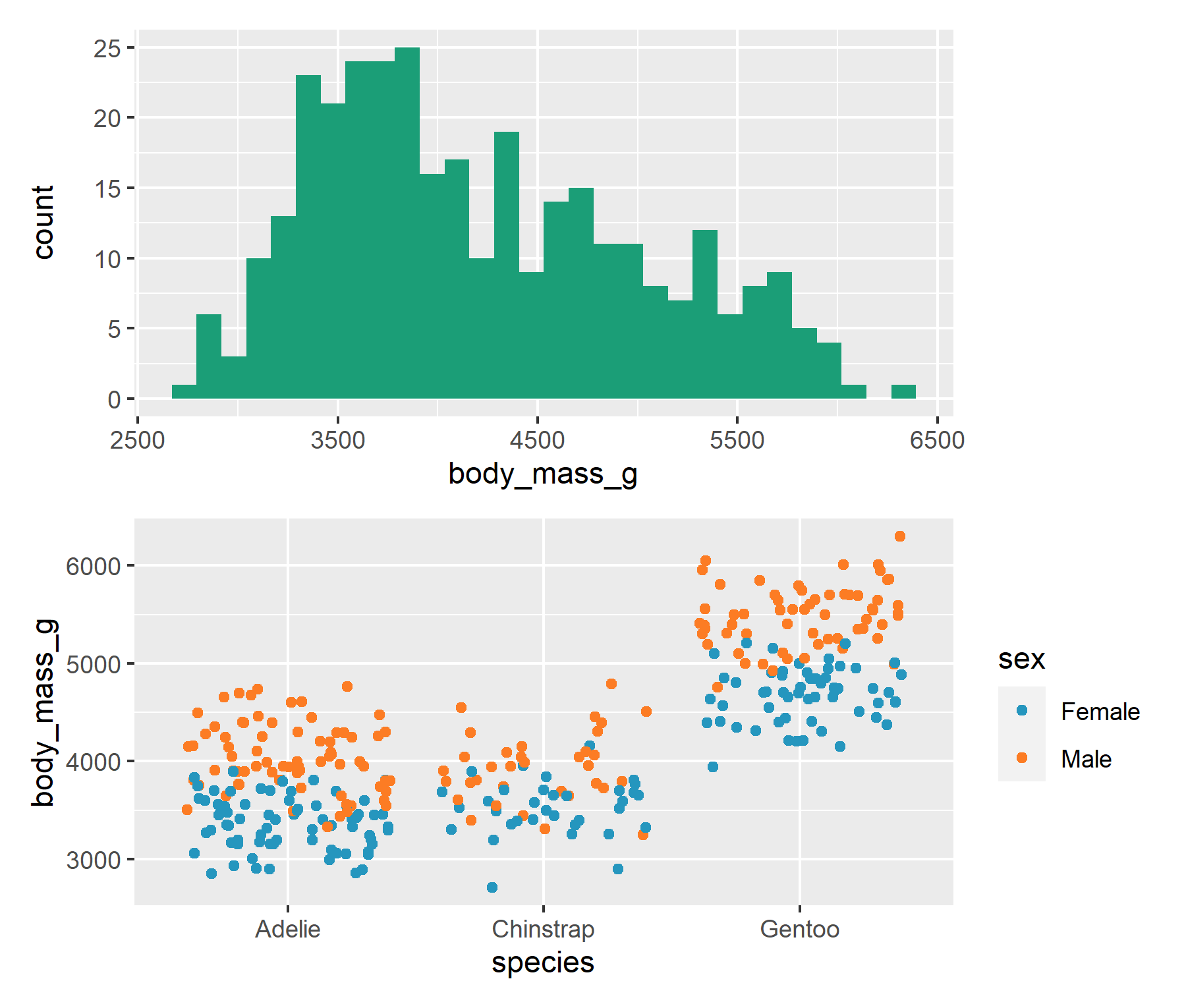``````# ggblanket
p1 <- penguins2 |>
gg_histogram(
x = body_mass_g,
pal = "#1b9e77")

p2 <- penguins2 |>
gg_jitter(
x = species,
y = body_mass_g,
col = sex,
pal = c("#2596be", "#fc7c24")
)

p1 / p2``````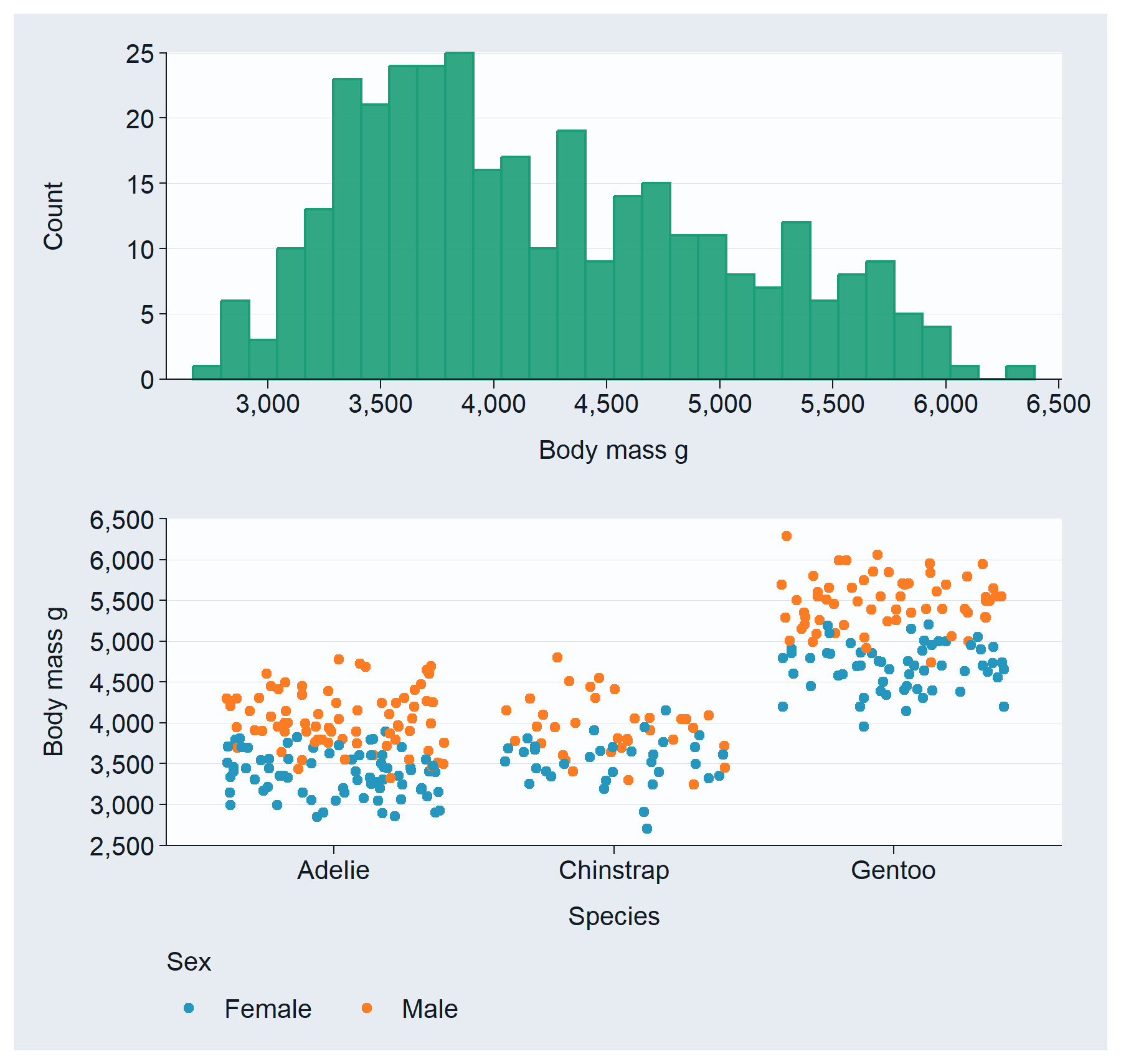### 4. A facet argument to facet by a variable

Faceting is treated as if it were an aesthetic. Users just provide an unquoted variable to facet by. If a single facet (or facet2) variable is provided, it’ll default to a “wrap” layout. But users can change this with a `facet_layout = "grid"` argument.

``````# ggplot2
penguins2 |>
ggplot() +
geom_violin(aes(x = sex,
y = body_mass_g)) +
facet_wrap(vars(species)) ``````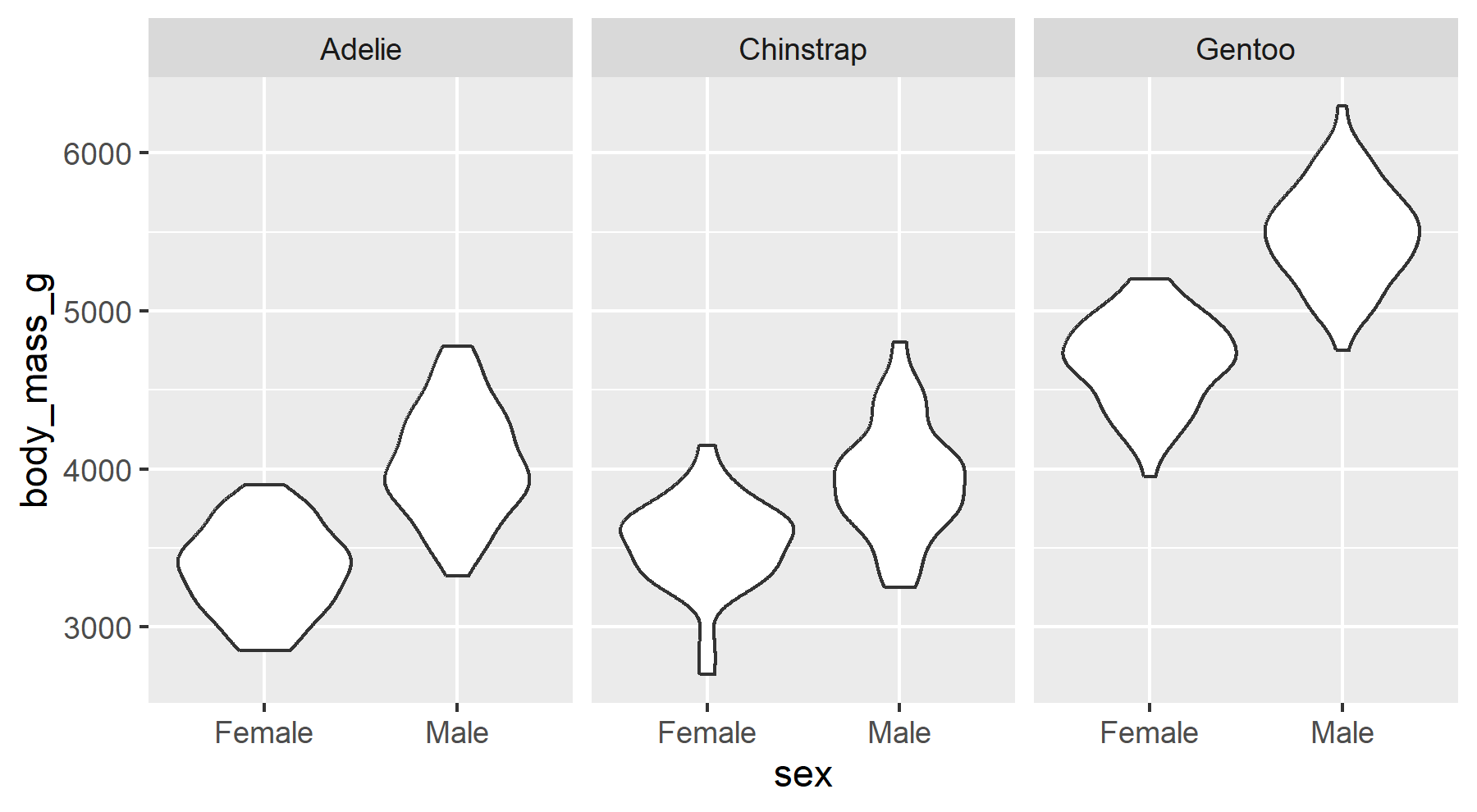``````# ggblanket
penguins2 |>
gg_violin(
x = sex,
y = body_mass_g,
facet = species)``````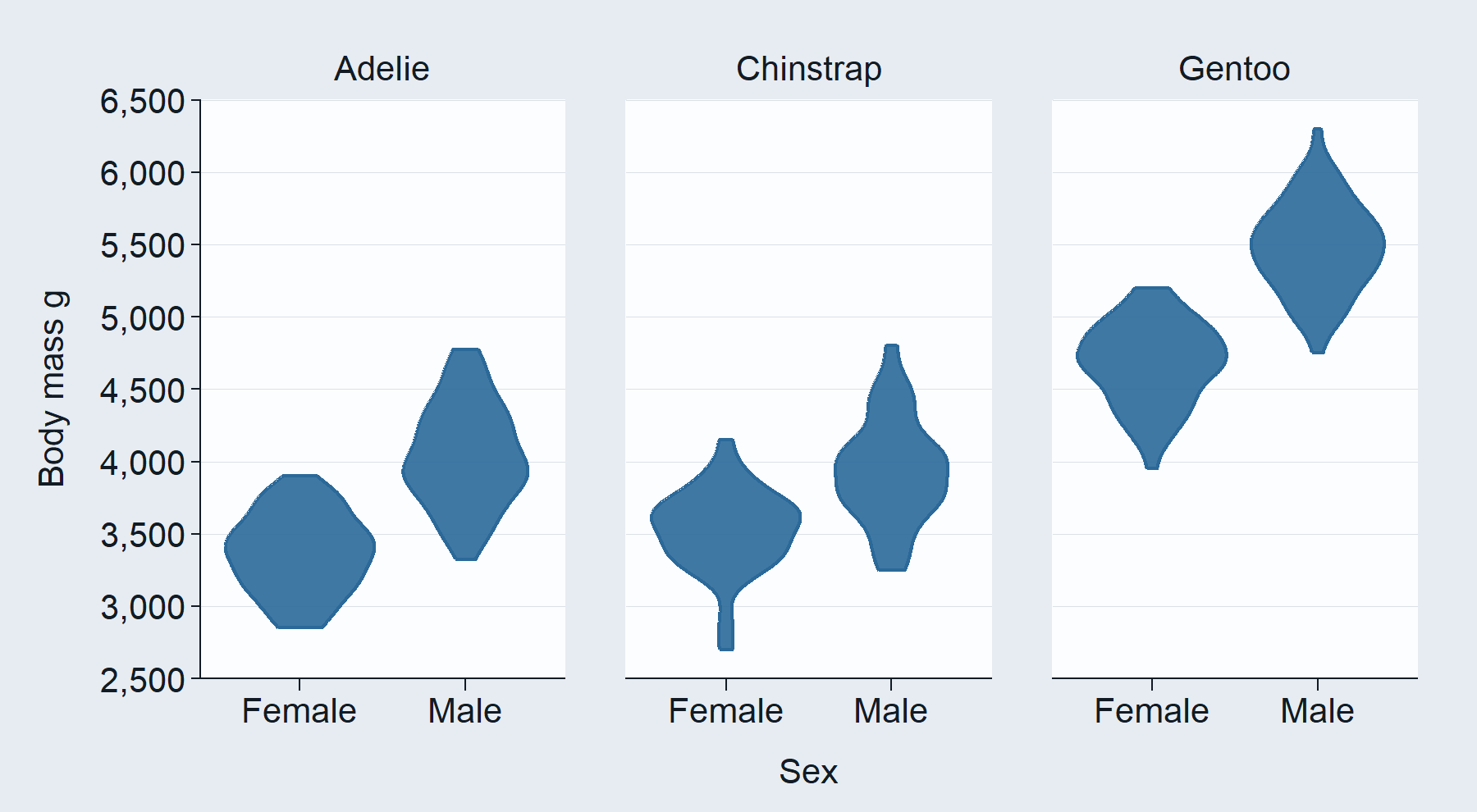### 5. An additional facet2 argument to facet by a 2nd variable

A `facet2` argument is also provided for extra functionality and flexibility. If both `facet`and `facet2` variables are provided, then it’ll default to a “grid” layout of `facet` by `facet2`. But users can change this with a `facet_layout = "wrap"` argument.

``````# ggplot2
penguins2 |>
ggplot() +
geom_histogram(aes(x = flipper_length_mm)) +
facet_grid(rows = vars(sex), cols = vars(species))``````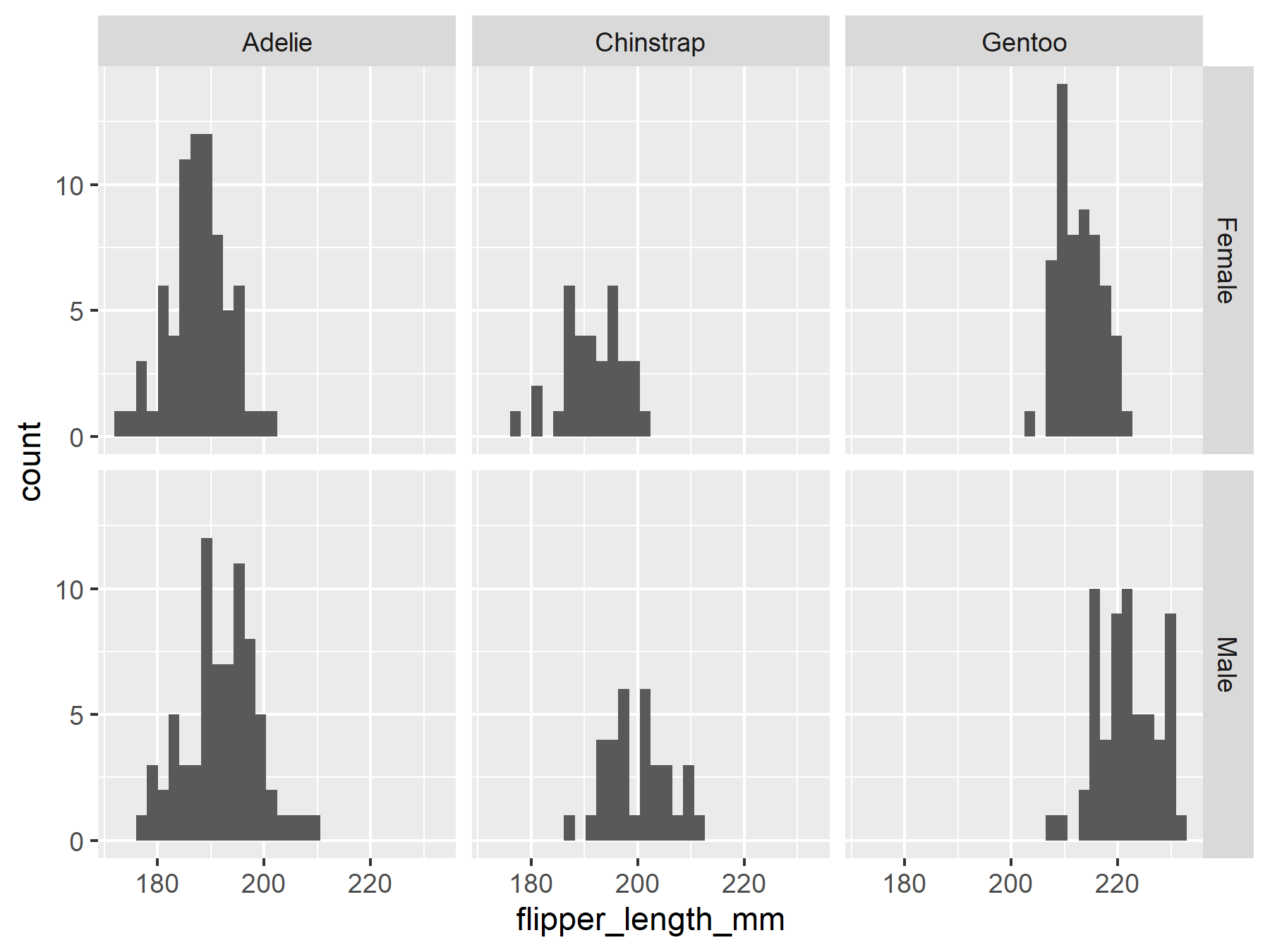``````# ggblanket
penguins2 |>
gg_histogram(
x = flipper_length_mm,
facet = species,
facet2 = sex)``````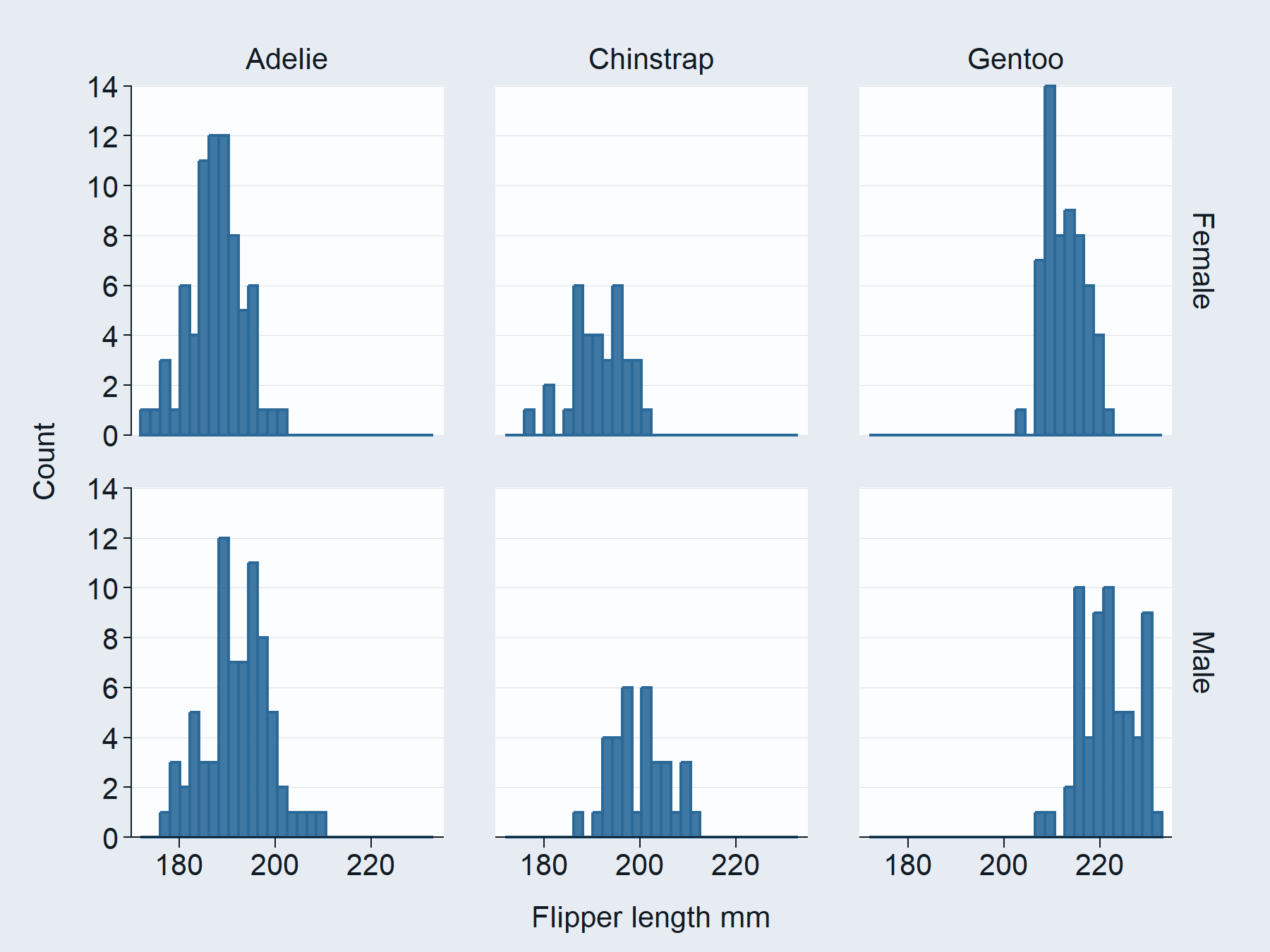### 6. Prefixed arguments to customise titles, scales, guides, and faceting

Prefixed arguments are available to customise titles, scales, guides, and faceting. These are designed to work with the Rstudio auto-complete to help users remember and find the adjustment they need. Users should first determine whether they want to change something that relates to x, y, col or facet. Then they should type this prefix and press the tab key to access the list of options from the Rstudio auto-complete. Then they can use the arrow keys, and press tab again to select what they want.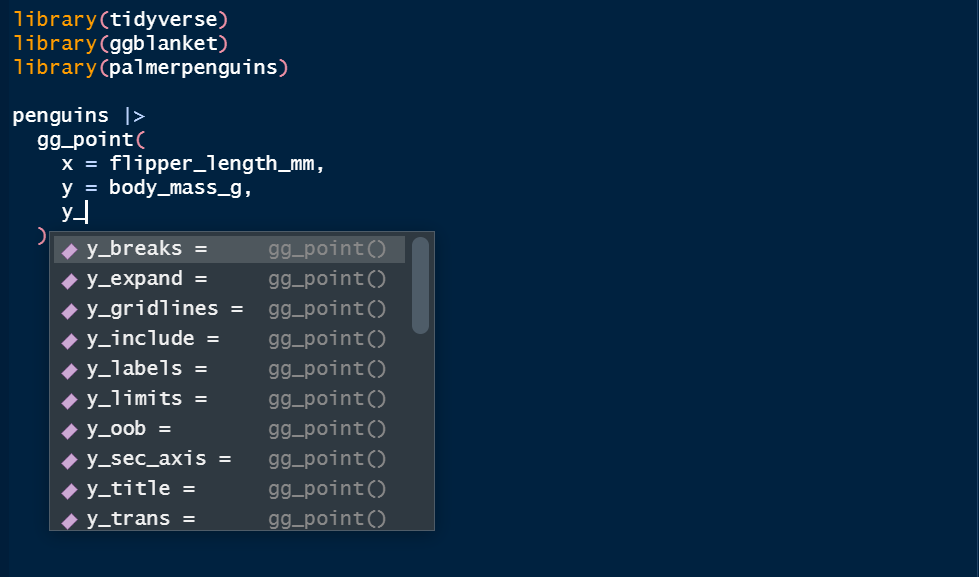``````# ggplot2
penguins2 |>
ggplot() +
geom_jitter(aes(x = species,
y = body_mass_g,
colour = sex)) +
expand_limits(y = 0) +
scale_x_discrete(labels = \(x) stringr::str_sub(x, 1, 1)) +
scale_y_continuous(breaks = scales::breaks_width(1500),
labels = scales::label_number(big.mark = " "),
expand = expansion(mult = c(0, 0.05)),
trans = "sqrt") +
labs(x = "Species", y = "Body mass (g)", col = NULL) +
theme(legend.position = "top") +
theme(legend.justification = "left") +
scale_colour_manual(values = scales::hue_pal()(2),
guide = ggplot2::guide_legend(title.position = "top"))``````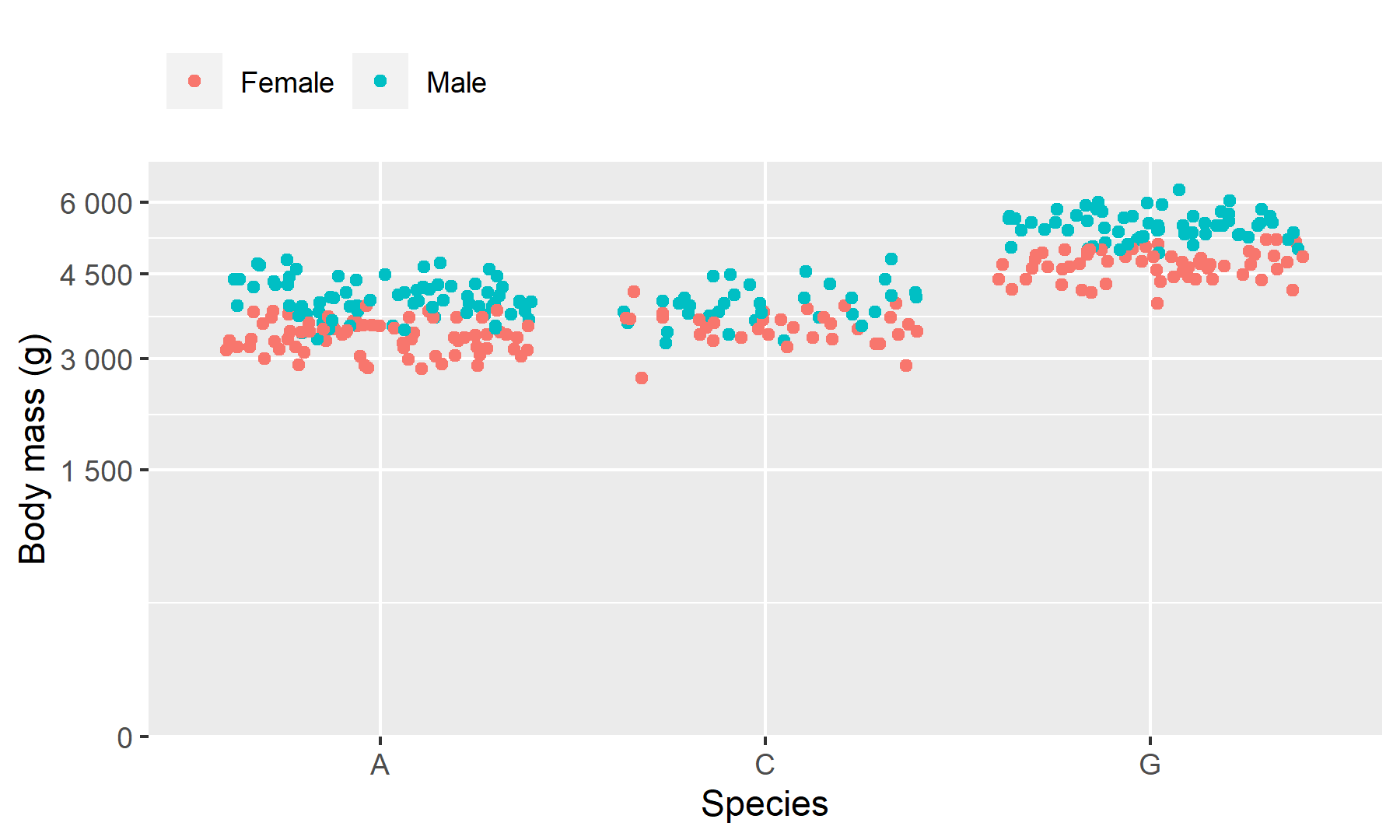``````# ggblanket
penguins2 |>
gg_jitter(
x = species,
y = body_mass_g,
col = sex,
x_labels = \(x) stringr::str_sub(x, 1, 1),
y_include = 0,
y_breaks = scales::breaks_width(1500),
y_labels = scales::label_number(big.mark = " "),
y_expand = expansion(mult = c(0, 0.05)),
y_trans = "sqrt",
y_title = "Body mass (g)",
col_legend_place = "t",
col_title = "")``````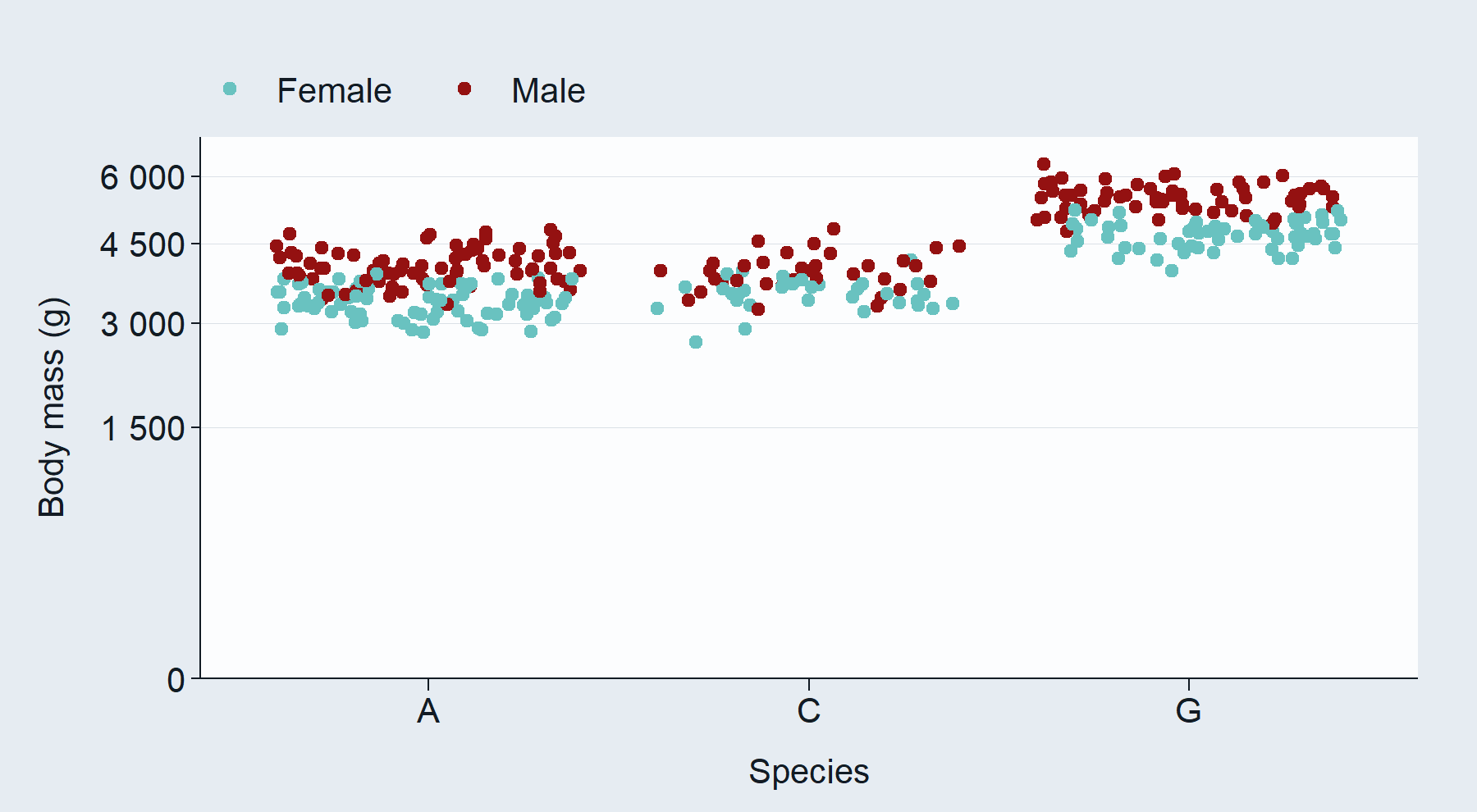### 7. Unspecified x/y/col titles converted to sentence case

Unspecified x/y/col titles are converted to sentence case with snakecase::to_sentence. All titles can be manually changed using the `*_title` arguments. The default conversion is intended to make titles sometimes able to be left as is.

``````# ggplot2
penguins2 |>
ggplot() +
geom_point(aes(x = flipper_length_mm,
y = body_mass_g,
colour = sex)) +
facet_wrap(vars(species)) +
scale_x_continuous(breaks = scales::breaks_pretty(n = 3)) ``````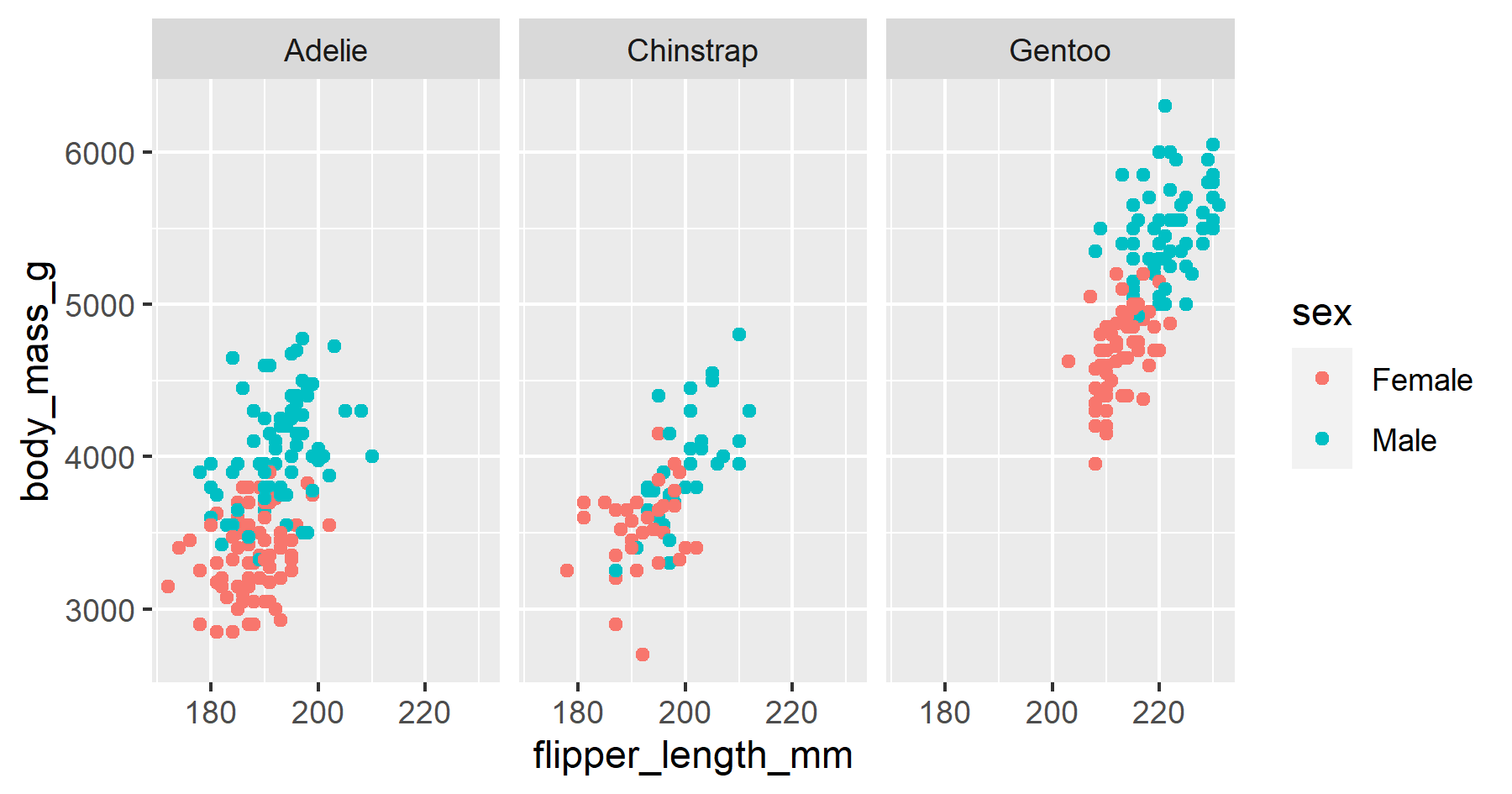``````# ggblanket
penguins2 |>
gg_point(
x = flipper_length_mm,
y = body_mass_g,
col = sex,
facet = species)``````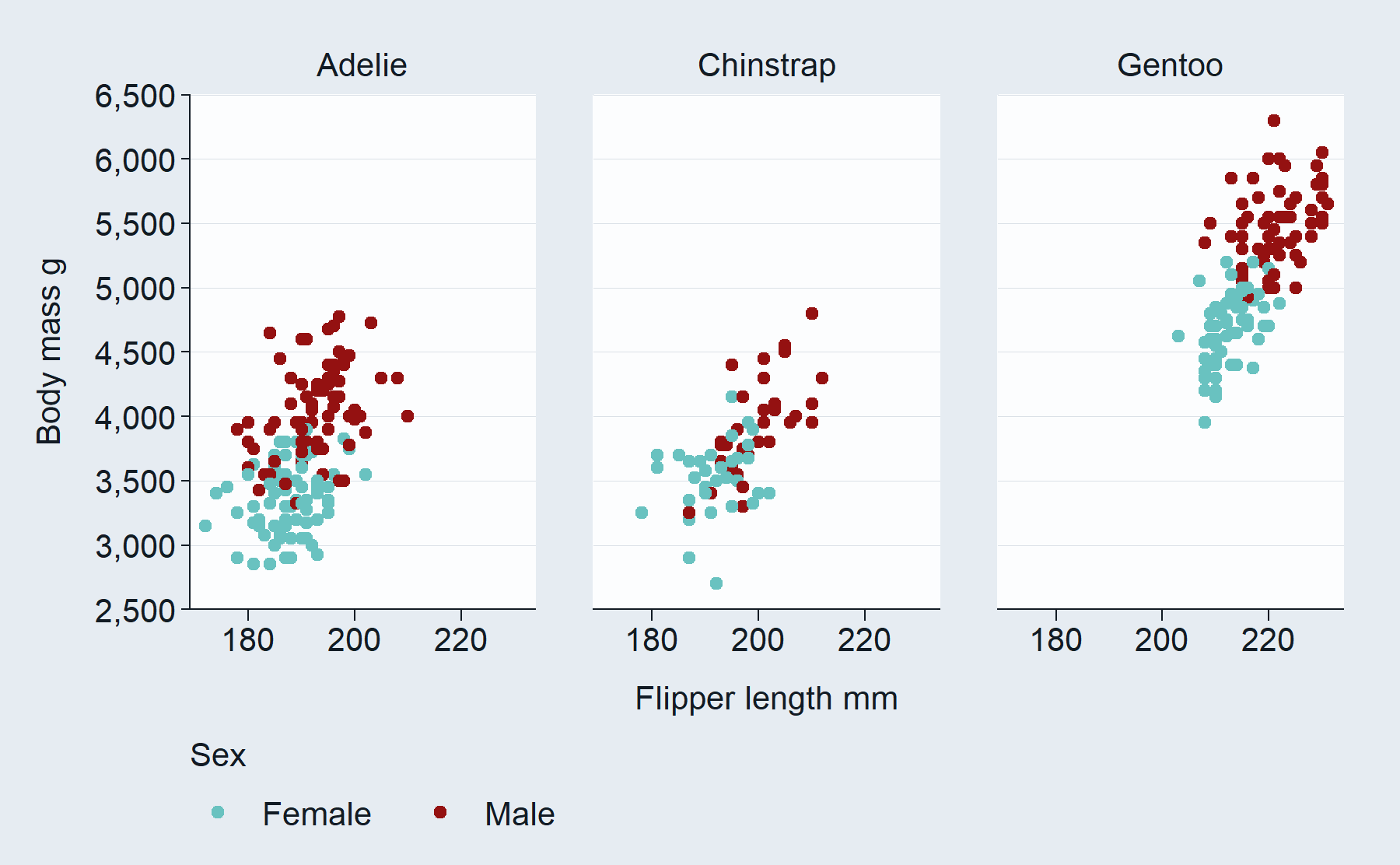### 8. Ability to set the theme, including to provided themes

The `ggplot2::theme_set` function can be used to set the theme globally, such that all subsequent plots will use this where the `theme` argument of a plot is left as NULL. Alternatively, users can use the `theme` argument in a `gg_*` function.

ggblanket provides two complete ggplot2 theme functions called `light_mode` (the default) and `dark_mode`. The first argument is the `base_size`. This changes the size of all the text to this, except the title is 10% higher and the caption is 10% lower. In quarto, it is likely that users will want to set the `*_mode` theme to have a larger `base_size` (e.g. `ggplot2::theme_set(light_mode(11))`).

Note that the `gg_*` function will control what gridlines are present and the placement of the legend - despite the theme provided. Therefore, if you are providing a theme other than `light_mode` or `dark_mode`, ggblanket works well if this theme has both vertical and horizontal gridlines. If users want everything adjusted as per the theme, then they can `+` their theme onto the plot instead.

Note that `theme_set(theme_grey())` resets the set theme for ggplot2 code to theme_grey and for ggblanket `gg_*` functions to `light_mode()`. If you want ggblanket `gg_*` functions to default to using `theme_grey()`, then you must modify the base_size slightly (e.g. `theme_set(theme_grey(11.01))`).

``````# ggblanket
theme_set(dark_mode())

penguins2 |>
gg_point(
x = flipper_length_mm,
y = body_mass_g,
col = sex,
title = "Penguins body mass by flipper length",
subtitle = "Palmer Archipelago, Antarctica",
caption = "Source: Gorman, 2020",
pal = c("#2596be", "#fc7c24"))``````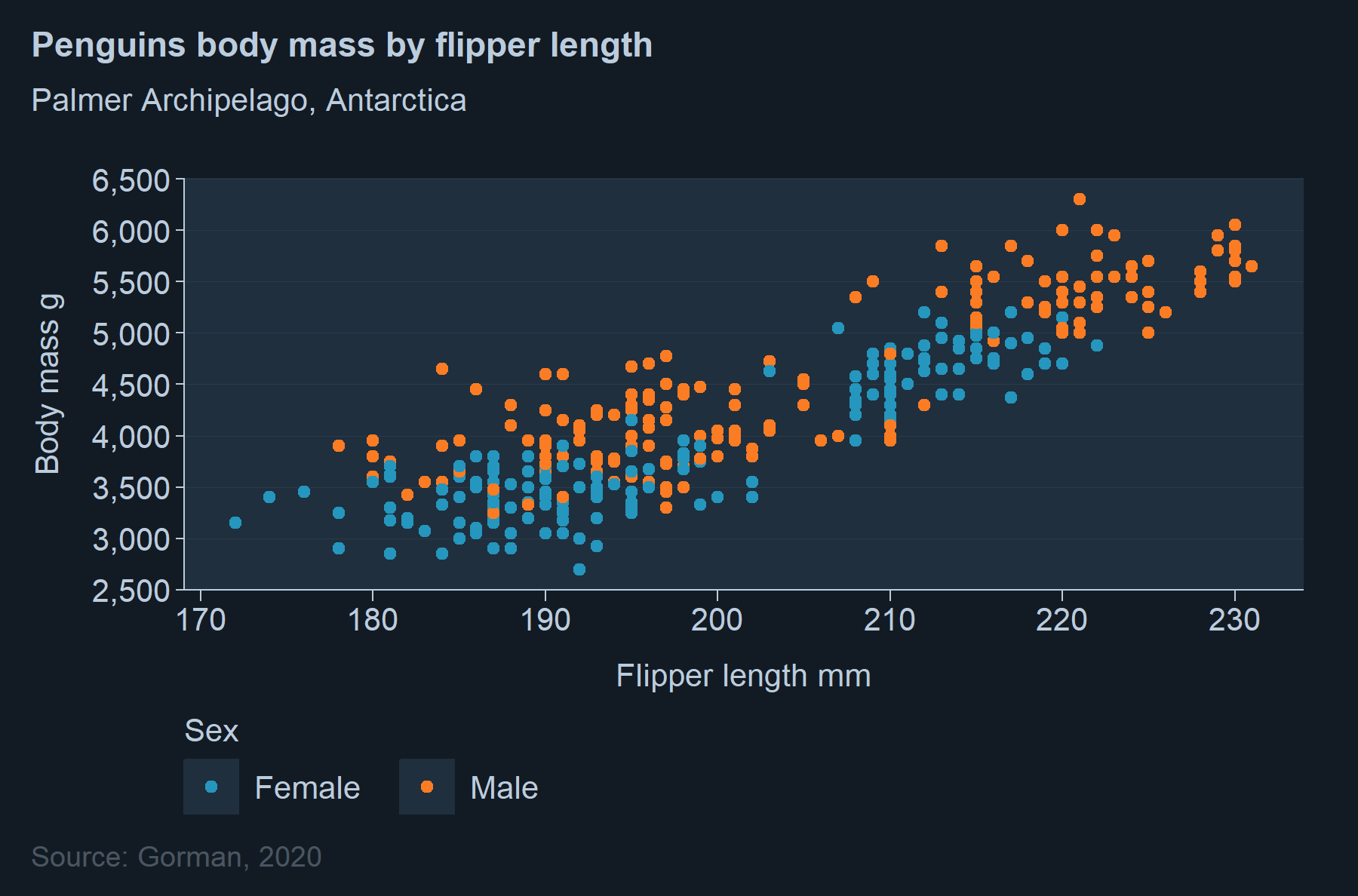``````# ggblanket
theme_set(light_mode(base_size = 12))

penguins2 |>
gg_point(
x = flipper_length_mm,
y = body_mass_g,
col = sex,
title = "Penguins body mass by flipper length",
subtitle = "Palmer Archipelago, Antarctica",
caption = "Source: Gorman, 2020",
pal = c("#2596be", "#fc7c24"))``````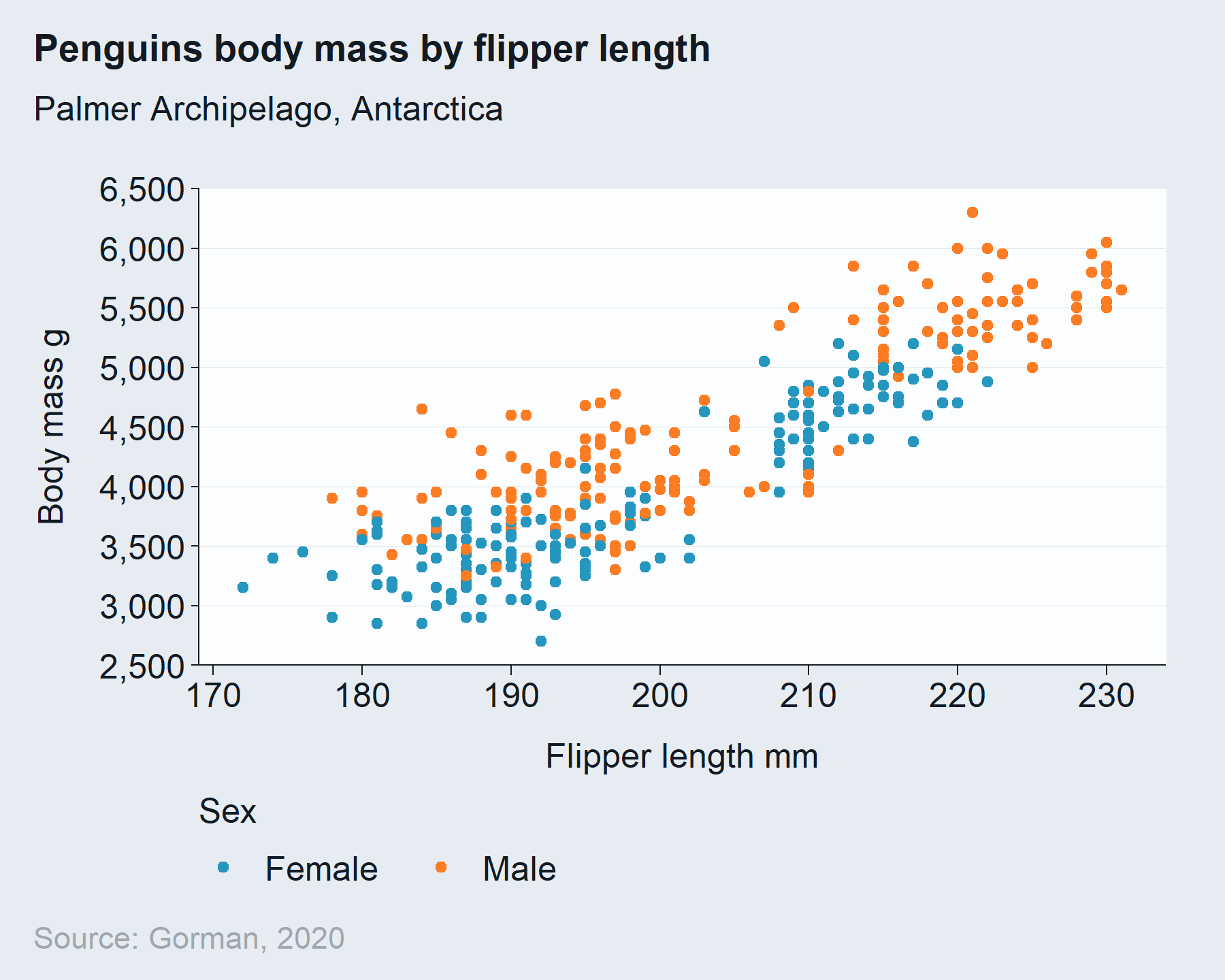``````
theme_set(theme_grey()) #unset the theme``````

### 9. Pretty defaults for symmetry

Where the orientation is normal (i.e. vertical):

• Default x scale limits and expanding are as per ggplot2 defaults
• Default numeric y scale limits (that are non-transformed) start and end on a break with zero expanding
• Default categorical (or numeric transformed) y scale limits and expanding are as per ggplot2 defaults
• Default removal of vertical gridlines

It does the opposite where the orientation is horizontal.

Note this symmetry approach does not apply: * if a transformation other than identity or reverse is applied to x or y scales. * for `gg_raster`, `gg_contour_filled` or `gg_density_2d_filled`

``````# ggplot2
penguins2 |>
group_by(species, sex) |>
summarise(body_mass_g = mean(body_mass_g)) |>
ggplot() +
geom_col(aes(x = body_mass_g,
y = species,
fill = sex),
position = "dodge",
width = 0.75)``````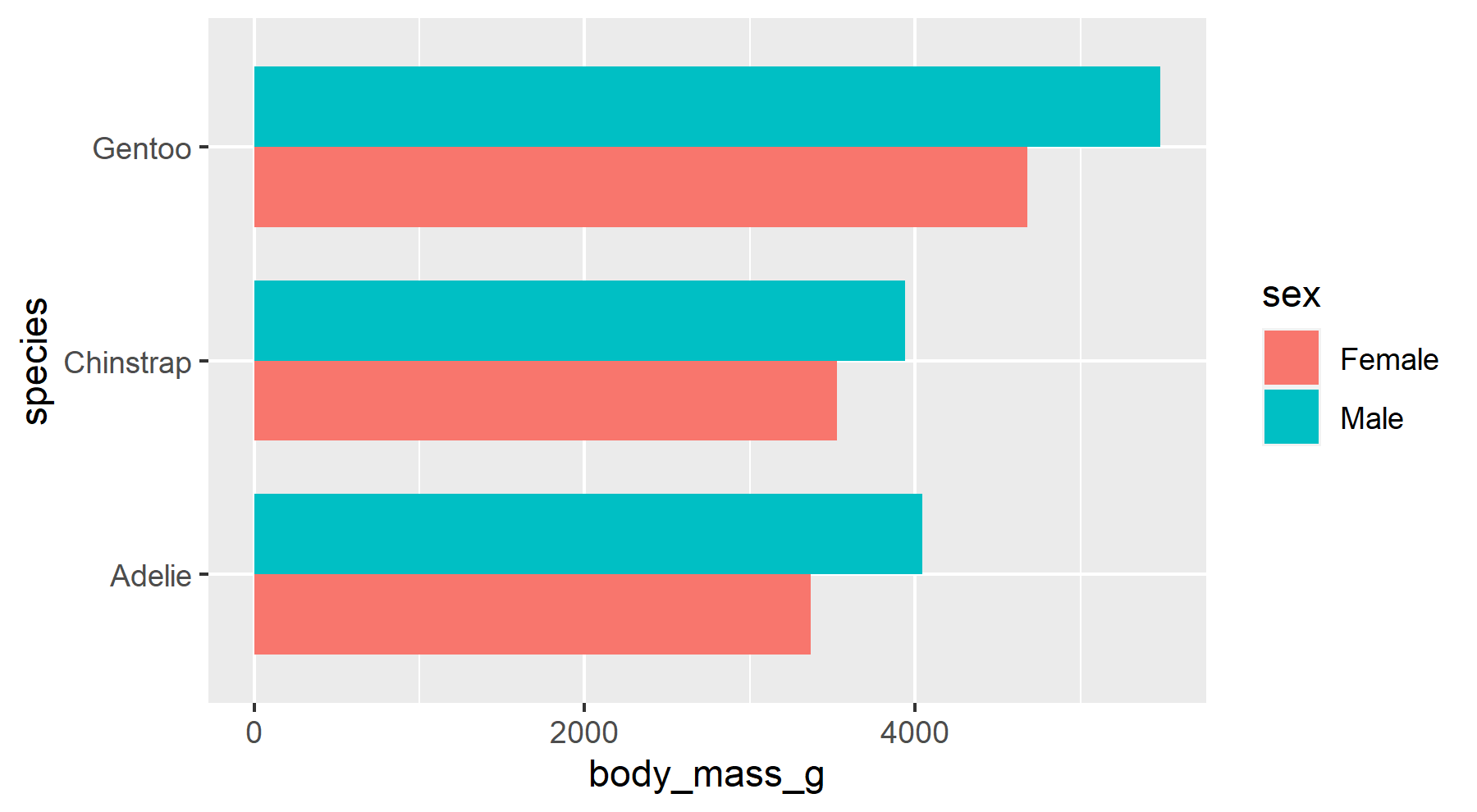``````# ggblanket
penguins2 |>
group_by(species, sex) |>
summarise(body_mass_g = mean(body_mass_g)) |>
gg_col(
x = body_mass_g,
y = species,
col = sex,
position = "dodge",
width = 0.75)``````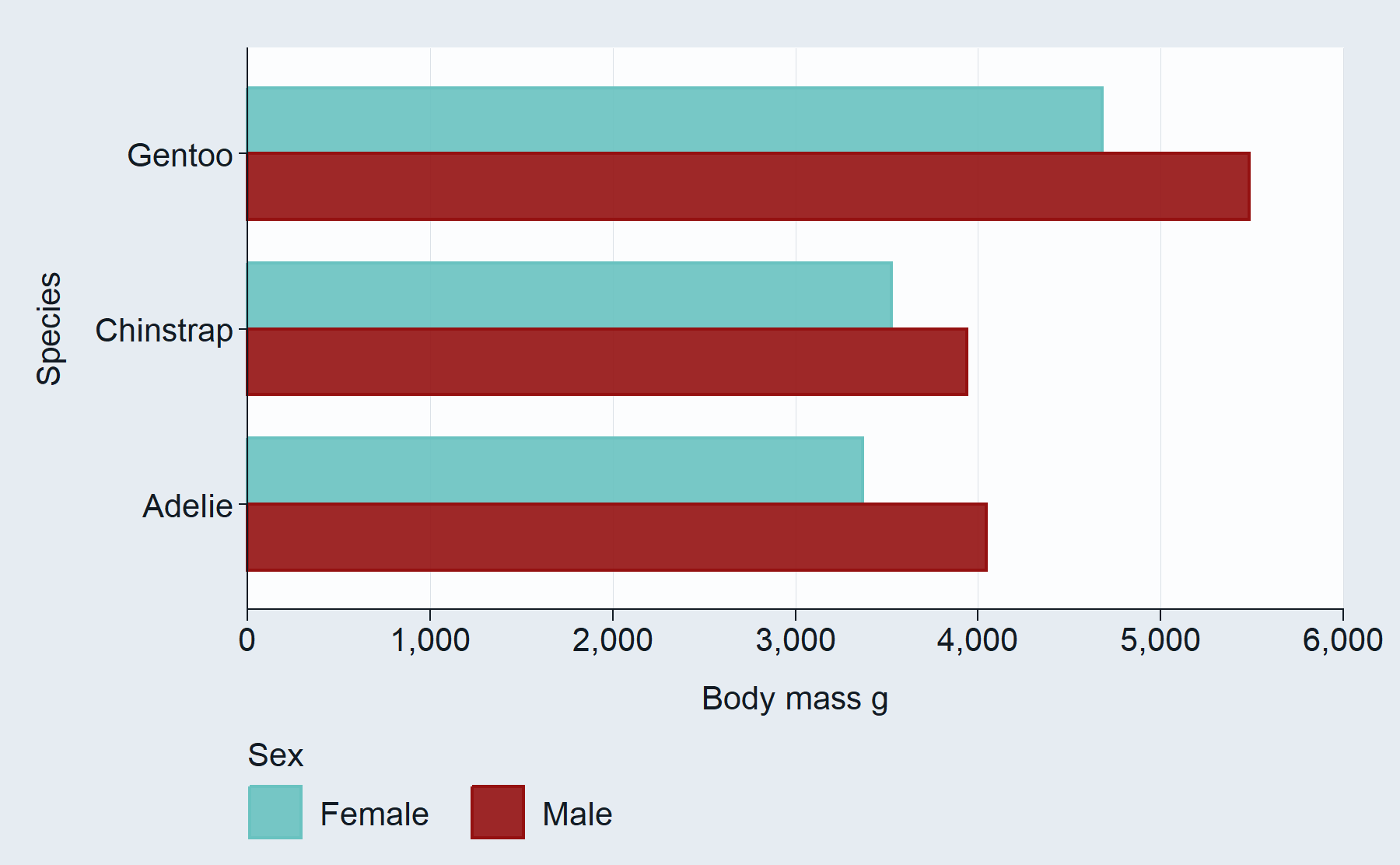### 10. Access to other geom_* arguments via `...`

The `...` argument is placed in the `gg_*` function within the wrapped `ggplot2::geom_*` function. This means all other arguments in the `geom_*` function are available to users. Common arguments from `...` to add are `size`, `linewidth` and `width`.

``````# ggblanket
penguins2 |>
gg_smooth(
x = flipper_length_mm,
y = body_mass_g,
col = sex,
linewidth = 0.5, #accessed via geom_smooth
level = 0.99) #accessed via geom_smooth``````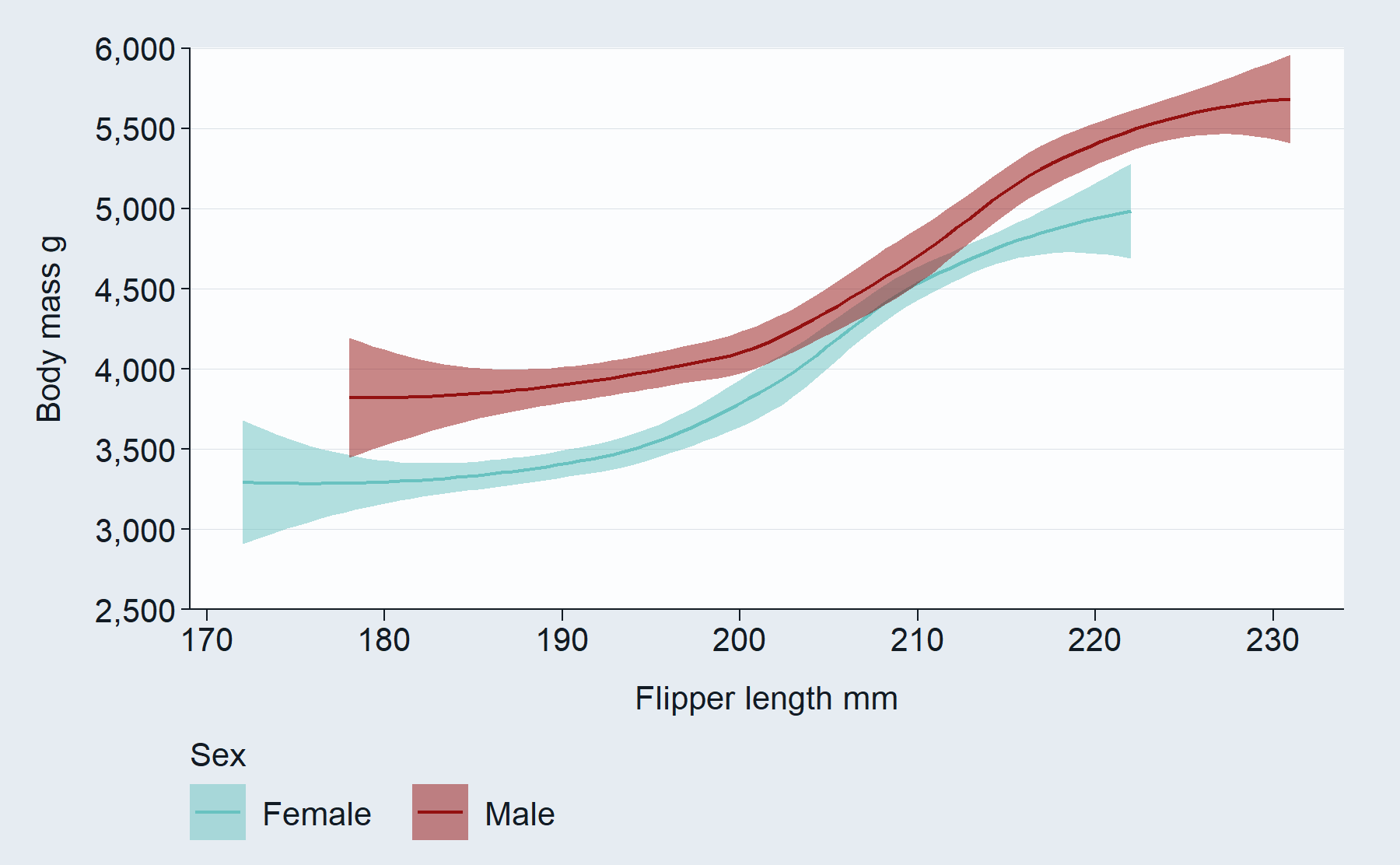### 11. Ability to add multiple geom_* layers

Users can make plots with multiple layers with ggblanket by adding on `ggplot2::geom_*` layers. The `gg_*` function puts the aesthetic variables (i.e. x, y, col) within the wrapped `ggplot` function. Therefore, these aesthetics will inherit to any subsequent layers added. Geoms will plot in order. The `gg_*` function will plot the associated geom as the first layer, and then other geoms will be plotted on top of it.

Users need to ensure that the scales built by their `gg_*` function are appropriate for subsequent layers. This is because the plot scales are constructed without knowledge of subsequent layers. They are built based on the `data`, `x`, `y`, `*_limits`, `*_include`, `facet_scales` and the underlying `stat` and `position` in the `gg_*` function. The `stat` and `position` is unique to each `geom_*` layer - it does not inherit.

Note that it is recommended that ggblanket users use the term `colour` within `geom_*` functions to help keep the distinction that the ggblanket `col` is the merged colour and fill.

``````# ggblanket + ggplot2
penguins2 |>
gg_boxplot(x = species,
y = body_mass_g,
width = 0.5,
outlier.colour = NA) +
geom_jitter(colour = pal_blue)``````